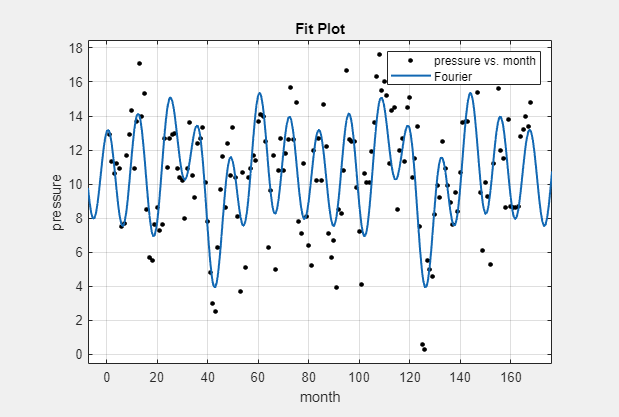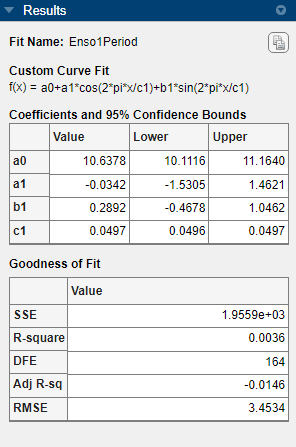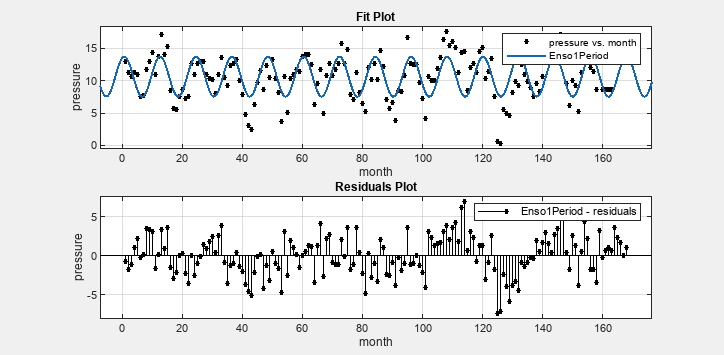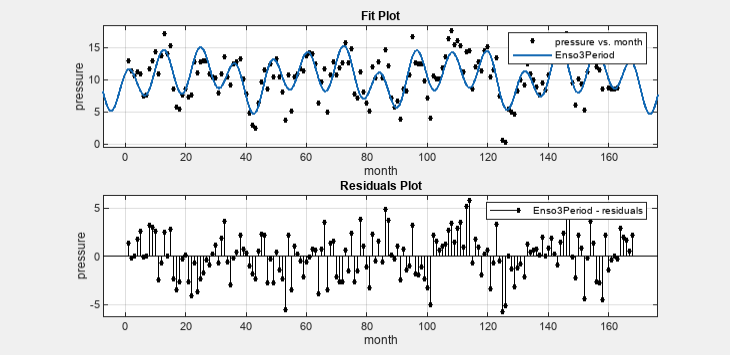## Custom Nonlinear ENSO Data Analysis

This example fits the ENSO data using several custom nonlinear equations. The ENSO data consists of monthly averaged atmospheric pressure differences between Easter Island and Darwin, Australia. This difference drives the trade winds in the southern hemisphere.

The ENSO data is clearly periodic, which suggests it can be described by a Fourier series:

`$y\left(x\right)={a}_{0}+\sum _{i=1}^{\infty }{a}_{i}\mathrm{cos}\left(2\pi \frac{x}{{c}_{i}}\right)+{b}_{i}\mathrm{sin}\left(2\pi \frac{x}{{c}_{i}}\right)$`

where ai and bi are the amplitudes, and ci are the periods (cycles) of the data. The question to answer here is how many cycles exist?

As a first attempt, assume a single cycle and fit the data using one cosine term and one sine term.

`${y}_{1}\left(x\right)={a}_{0}+{a}_{1}\mathrm{cos}\left(2\pi \frac{x}{{c}_{1}}\right)+{b}_{1}\mathrm{sin}\left(2\pi \frac{x}{{c}_{1}}\right)$`

If the fit does not describe the data well, add additional cosine and sine terms with unique period coefficients until a good fit is obtained.

The equation is nonlinear because an unknown coefficient c1 is included as part of the trigonometric function arguments.

### Load Data and Fit Library and Custom Fourier Models

1. Load the data and open the Curve Fitting app:

```load enso cftool```

2. The toolbox includes the Fourier series as a nonlinear library equation. However, the library equation does not meet the needs of this example because its terms are defined as fixed multiples of the fundamental frequency w. Refer to Fourier Series for more information. Create the built-in library Fourier fit to compare with your custom equations:

1. Select `month` for X data and `pressure` for Y data.

2. Select `Fourier` for the model type.

3. Enter `Fourier` for the Fit name.

4. Change the number of terms to `8`.

Observe the library model fit. In the next steps you will create custom equations to compare.3. Duplicate your fit. Right-click your fit in the Table of Fits and select Duplicate ‘Fourier’.

4. Name the new fit `Enso1Period`.

5. Change the fit type from `Fourier` to `Custom Equation`.

6. Replace the example text in the equation edit box with

`a0+a1*cos(2*pi*x/c1)+b1*sin(2*pi*x/c1)`The toolbox applies the fit to the `enso` data.

The graphical and numerical results shown here indicate that the fit does not describe the data well. In particular, the fitted value for `c1` is unreasonably small. Your initial fit results might differ from these results because the starting points are randomly selected.By default, the coefficients are unbounded and have random starting values from 0 to 1. The data include a periodic component with a period of about 12 months. However, with `c1` unconstrained and with a random starting point, this fit failed to find that cycle.

### Use Fit Options to Constrain a Coefficient

1. To assist the fitting procedure, constrain `c1` to a value from 10 to 14. Click the button to view and edit constraints for unknown coefficients.

2. In the Fit Options dialog box, observe that by default the coefficients are unbounded (bounds of `-Inf` and `Inf`).

3. Change the Lower and Upper bounds for `c1` to constrain the cycle from 10 to 14 months, as shown next.4. Click . The Curve Fitting app refits.

5. Observe the new fit and the residuals plot. If necessary, select View > Residuals Plot or use the toolbar button.The fit appears to be reasonable for some data points but clearly does not describe the entire data set very well. As predicted, the numerical results in the Results pane (`c1=11.94`) indicate a cycle of approximately 12 months. However, the residuals show a systematic periodic distribution, indicating that at least one more cycle exists. There are additional cycles that you should include in the fit equation.

### Create Second Custom Fit with Additional Terms and Constraints

To refine your fit, you need to add an additional sine and cosine term to y1(x) as follows:

`${y}_{2}\left(x\right)={y}_{1}\left(x\right)+{a}_{2}\mathrm{cos}\left(2\pi \frac{x}{{c}_{2}}\right)+{b}_{2}\mathrm{sin}\left(2\pi \frac{x}{{c}_{2}}\right)$`

and constrain the upper and lower bounds of c2 to be roughly twice the bounds used for c1.

1. Duplicate your fit by right-clicking it in the Table of Fits and selecting Duplicate ‘Enso1Period’.

2. Name the new fit `Enso2Period`.

3. Add these terms to the end of the previous equation:

`+a2*cos(2*pi*x/c2)+b2*sin(2*pi*x/c2)`

4. Click . When you edit the custom equation, the tool remembers your fit options. Observe the Lower and Upper bounds for `c1` still constrain the cycle from 10 to 14 months. Add more fit options:

1. Change the Lower and Upper for `c2` to be roughly twice the bounds used for `c1` (20<`c2`<30).

2. Change the StartPoint for `a0` to `5`.

As you change each setting, the Curve Fitting app refits. The fit and residuals are shown next.The fit appears reasonable for most data points. However, the residuals indicate that you should include another cycle to the fit equation.

### Create a Third Custom Fit with Additional Terms and Constraints

As a third attempt, add an additional sine and cosine term to y2(x)

`${y}_{3}\left(x\right)={y}_{2}\left(x\right)+{a}_{3}\mathrm{cos}\left(2\pi \frac{x}{{c}_{3}}\right)+{b}_{3}\mathrm{sin}\left(2\pi \frac{x}{{c}_{3}}\right)$`

and constrain the lower bound of c3 to be roughly triple the value of c1.

1. Duplicate your fit by right-clicking it in the Table of Fits and selecting Duplicate ‘Enso2Period’.

2. Name the new fit `Enso3Period`.

3. Add these terms to the end of the previous equation:

`+a3*cos(2*pi*x/c3)+b3*sin(2*pi*x/c3)`

4. Click Observe your previous fit options are still present.

1. Change the bound for `c3` to be `36`, which is roughly triple the value of `c1`.2. Close the dialog box. The Curve Fitting app refits. The fit and residuals appear next.The fit is an improvement over the previous two fits, and appears to account for most of the cycles in the ENSO data set. The residuals appear random for most of the data, although a pattern is still visible indicating that additional cycles might be present, or you can improve the fitted amplitudes.

In conclusion, Fourier analysis of the data reveals three significant cycles. The annual cycle is the strongest, but cycles with periods of approximately 44 and 22 months are also present. These cycles correspond to El Nino and the Southern Oscillation (ENSO).